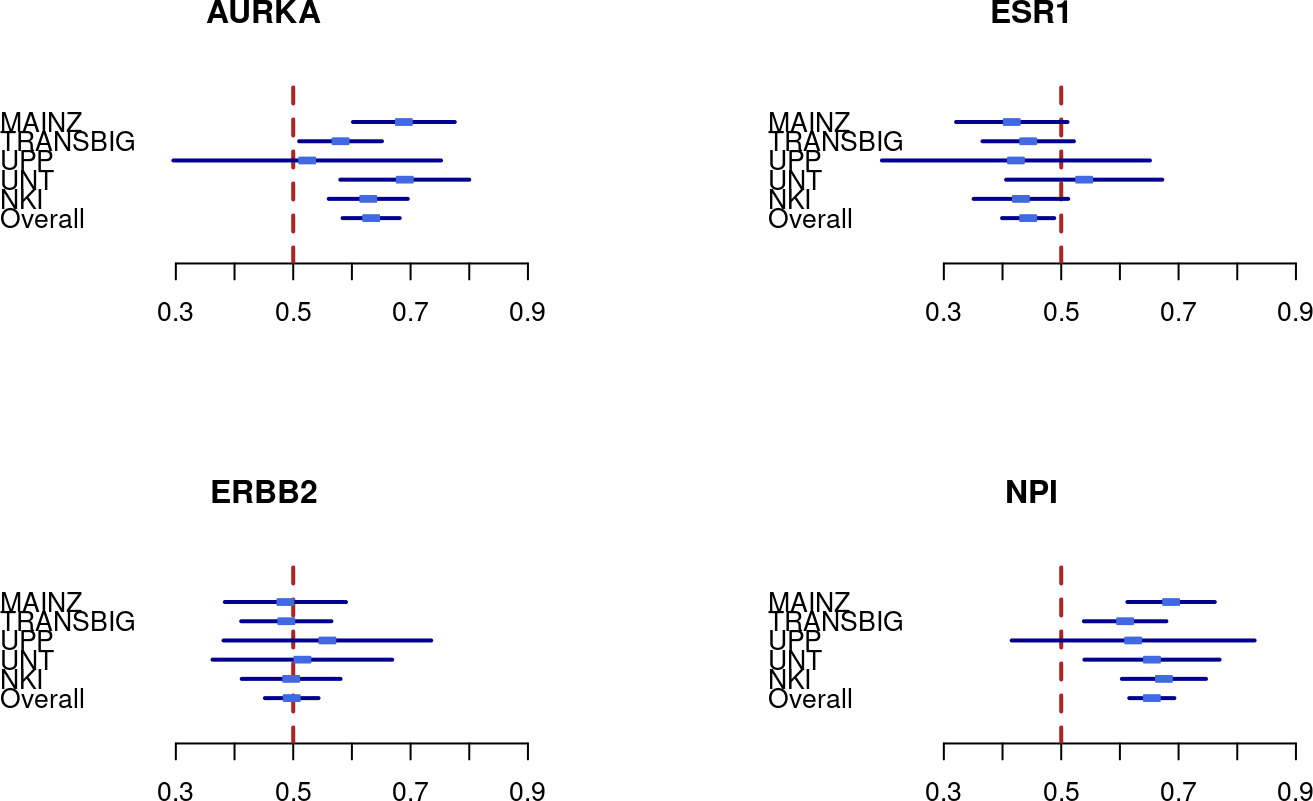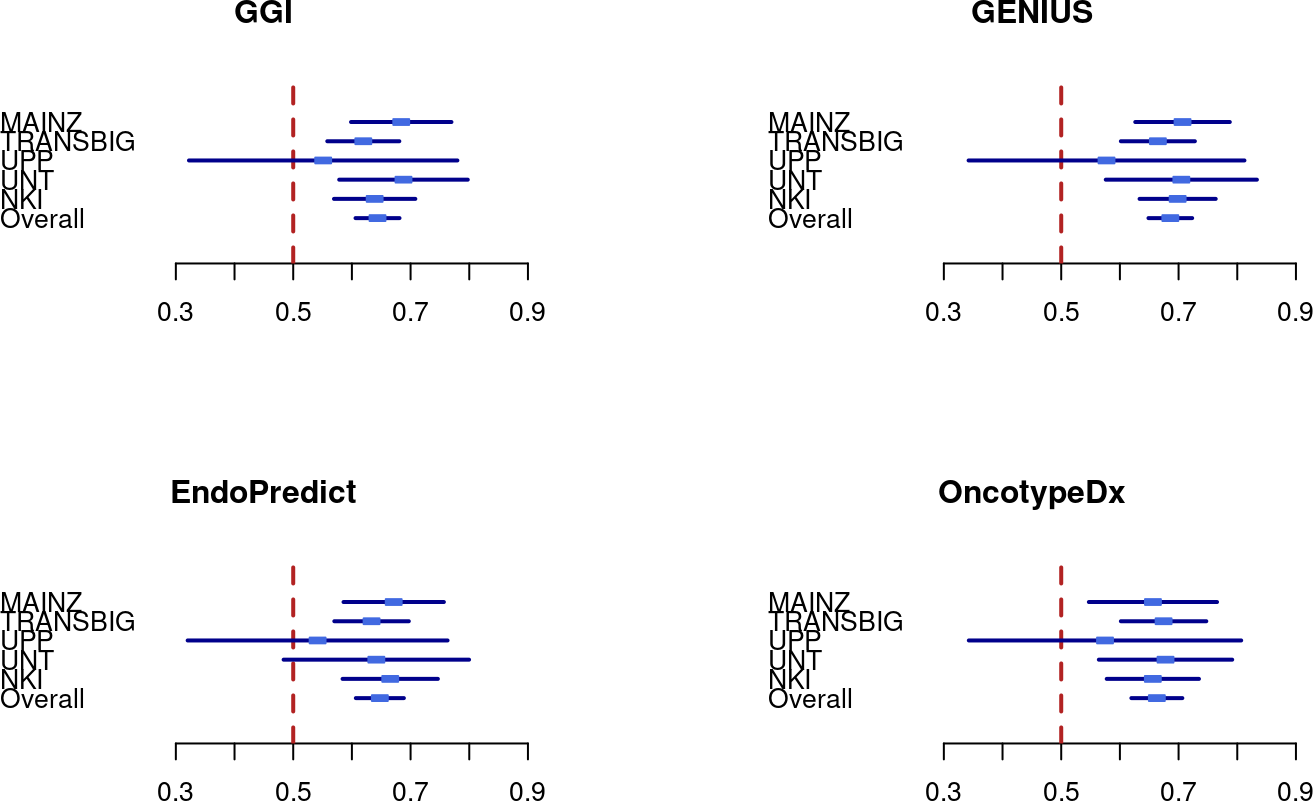# 1 Introduction

The genefu package is providing relevant functions for gene expression analysis, especially in breast cancer. This package includes a number of algorithms for molecular subtype classification. The package also includes implementations of prognostic prediction algorithms, along with lists of prognostic gene signatures on which these algorithms were based.

Please refer to the manuscript URL and Lab website: http://www.pmgenomics.ca/bhklab/software/genefu

Please also refer to the References section below, for additional information on publications that have cited Version 1 of genefu.

First we load the genefu into the workspace. The package is publicly available and can be installed from Bioconductor version 2.8 or higher in R version 2.13.0 or higher.

To install the genefu package:

``BiocManager::install("genefu")``

For computing the risk scores, estimates of the performance of the risk scores, combining the estimates and comparing the estimates we have to load the `genefu` and `survcomp` packages into the workspace. We also load all the packages we need to conduct the case studies.

``````library(genefu)
library(xtable)
library(rmeta)
library(Biobase)
library(caret)``````

# 3 Load Datasets and Packages for Case Studies

The following case study compares risk prediction models. This includes computing risk scores, computing estimates of the performance of the risk scores, as well as combining the estimates and comparing them.

The five data sets that we use in the case study are publicly available as experimental data packages on Bioconductor.org. In particular we used:

Please Note: We don’t use the breastCancerVDX experimental package in this case study since it has been used as training data set for GENIUS. Please refer to Haibe-Kains et al, 2010. The breastCancerVDX is found at the following link:

These experimental data packages can be installed from Bioconductor version 2.8 or higher in R version 2.13.0 or higher. For the experimental data packages the commands for installing the data sets are:

``````BiocManager::install("breastCancerMAINZ")
BiocManager::install("breastCancerTRANSBIG")
BiocManager::install("breastCancerUPP")
BiocManager::install("breastCancerUNT")
BiocManager::install("breastCancerNKI")``````
``````library(breastCancerMAINZ)
library(breastCancerTRANSBIG)
library(breastCancerUPP)
library(breastCancerUNT)
library(breastCancerNKI)``````

Table 1: Detailed overview for the data sets used in the case study.
Dataset Patients [#] ER+ [#] HER2+ [#] Age [years] Grade [1/2/3] Platform
MAINZ 200 155 23 25-90 29/136/35 HGU133A
TRANSBIG 198 123 35 24-60 30/83/83 HGU133A
UPP 251 175 46 28-93 67/128/54 HGU133AB
UNT 137 94 21 24-73 32/51/29 HGU133AB
NKI 337 212 53 26-62 79/109/149 Agilent
Overall 1123 759 178 25-73 237/507/350 Affy/Agilent

Table 1 shows an overview of the data sets and the patients (n=1123). Information on ER and HER2 status, as well as patient ages (range of patient ages per dataset) has been extracted from the phenotype (pData) of the corresponding dataset under the Gene Expression Omnibus (GEO). The corresponding GEO accession numbers of the Mainz, Transbig, UPP, and UNT datasets are GSE11121,GSE7390,GSE3494, and GSE2990 respectively. Data was also obtained from the publication supplementary information for the NKI dataset.

For analysis involving molecular subtyping classifications [Section 4], we perform molecular subtyping on each of the datasets seperately, after the removal of duplicate patients in the datasets.

For analysis comparing risk prediction models and determining prognosis [Section 5], we selected from those 1123 breast cancer patients only the patients that are node negative and didn’t receive any treatment (except local radiotherapy), which results in 713 patients [please consult Section 5 for more details] .

``````data(breastCancerData)
cinfo <- colnames(pData(mainz7g))
data.all <- c("transbig7g"=transbig7g, "unt7g"=unt7g, "upp7g"=upp7g,
"mainz7g"=mainz7g, "nki7g"=nki7g)

idtoremove.all <- NULL
duplres <- NULL

## No overlaps in the MainZ and NKI datasets.

## Focus on UNT vs UPP vs TRANSBIG
demo.all <- rbind(pData(transbig7g), pData(unt7g), pData(upp7g))
dn2 <- c("TRANSBIG", "UNT", "UPP")

## Karolinska
## Search for the VDXKIU, KIU, UPPU series
ds2 <- c("VDXKIU", "KIU", "UPPU")
demot <- demo.all[complete.cases(demo.all[ , c("series")]) &
is.element(demo.all[ , "series"], ds2), ]

# Find the duplicated patients in that series
duplid <- sort(unique(demot[duplicated(demot[ , "id"]), "id"]))
duplrest <- NULL
for(i in 1:length(duplid)) {
tt <- NULL
for(k in 1:length(dn2)) {
myx <- sort(row.names(demot)[complete.cases(demot[ , c("id", "dataset")]) &
demot[ , "id"] == duplid[i] &
demot[ , "dataset"] == dn2[k]])
if(length(myx) > 0) { tt <- c(tt, myx) }
}
duplrest <- c(duplrest, list(tt))
}
names(duplrest) <- duplid
duplres <- c(duplres, duplrest)

## Oxford
## Search for the VVDXOXFU, OXFU series
ds2 <- c("VDXOXFU", "OXFU")
demot <- demo.all[complete.cases(demo.all[ , c("series")]) &
is.element(demo.all[ , "series"], ds2), ]

# Find the duplicated patients in that series
duplid <- sort(unique(demot[duplicated(demot[ , "id"]), "id"]))
duplrest <- NULL
for(i in 1:length(duplid)) {
tt <- NULL
for(k in 1:length(dn2)) {
myx <- sort(row.names(demot)[complete.cases(demot[ , c("id", "dataset")]) &
demot[ , "id"] == duplid[i] &
demot[ , "dataset"] == dn2[k]])
if(length(myx) > 0) { tt <- c(tt, myx) }
}
duplrest <- c(duplrest, list(tt))
}
names(duplrest) <- duplid
duplres <- c(duplres, duplrest)

## Full set duplicated patients
duPL <- sort(unlist(lapply(duplres, function(x) { return(x[-1]) } )))``````

# 4 Case Studies

## 4.1 Compare Molecular Subtype Classifications

We now perform molecular subtyping on each of the datasets. Here, we perform subtyping using the PAM50 as well as the SCMOD2 subtyping algorithms.

``````# Load the requisite data
data(scmod2.robust)
data(pam50.robust)
data(scmgene.robust)
data(sig.ggi)
data(scmod1.robust)
data(sig.genius)

dn <- c("transbig", "unt", "upp", "mainz", "nki")
dn.platform <- c("affy", "affy", "affy", "affy", "agilent")
res <- ddemo.all <- ddemo.coln <- NULL

for(i in 1:length(dn)) {

dd <- get(data(list=dn[i]))
#Remove duplicates identified first
message("obtained dataset!")

#Extract expression set, pData, fData for each dataset
ddata <- t(exprs(dd))

ddemo <- phenoData(dd)@data

if(length(intersect(rownames(ddata),duPL))>0)
{
ddata<-ddata[-which(rownames(ddata) %in% duPL),]
ddemo<-ddemo[-which(rownames(ddemo) %in% duPL),]
}

dannot <- featureData(dd)@data

# MOLECULAR SUBTYPING
# Perform subtyping using scmod2.robust
# scmod2.robust: List of parameters defining the subtype clustering model
# (as defined by Wirapati et al)

# OBSOLETE FUNCTION CALL - OLDER VERSIONS OF GENEFU
# SubtypePredictions<-subtype.cluster.predict(sbt.model=scmod2.robust,data=ddata,
#                                               annot=dannot,do.mapping=TRUE,
#                                               verbose=TRUE)

# CURRENT FUNCTION CALL - NEWEST VERSION OF GENEFU
SubtypePredictions <- molecular.subtyping(sbt.model = "scmod2",data = ddata,
annot = dannot,do.mapping = TRUE)

#Get sample counts pertaining to each subtype
table(SubtypePredictions\$subtype)
#Select samples pertaining to Basal Subtype
Basals<-names(which(SubtypePredictions\$subtype == "ER-/HER2-"))
#Select samples pertaining to HER2 Subtype
HER2s<-names(which(SubtypePredictions\$subtype == "HER2+"))
#Select samples pertaining to Luminal Subtypes
LuminalB<-names(which(SubtypePredictions\$subtype == "ER+/HER2- High Prolif"))
LuminalA<-names(which(SubtypePredictions\$subtype == "ER+/HER2- Low Prolif"))

#ASSIGN SUBTYPES TO EVERY SAMPLE, ADD TO THE EXISTING PHENODATA
ddemo\$SCMOD2<-SubtypePredictions\$subtype
ddemo[LuminalB,]\$SCMOD2<-"LumB"
ddemo[LuminalA,]\$SCMOD2<-"LumA"
ddemo[Basals,]\$SCMOD2<-"Basal"
ddemo[HER2s,]\$SCMOD2<-"Her2"

# Perform subtyping using PAM50
# Matrix should have samples as ROWS, genes as COLUMNS
# rownames(dannot)<-dannot\$probe<-dannot\$EntrezGene.ID

# OLDER FUNCTION CALL
# PAM50Preds<-intrinsic.cluster.predict(sbt.model=pam50,data=ddata,
#                                         annot=dannot,do.mapping=TRUE,
#                                         verbose=TRUE)

PAM50Preds<-molecular.subtyping(sbt.model = "pam50",data=ddata,
annot=dannot,do.mapping=TRUE)

table(PAM50Preds\$subtype)
ddemo\$PAM50<-PAM50Preds\$subtype
LumA<-names(PAM50Preds\$subtype)[which(PAM50Preds\$subtype == "LumA")]
LumB<-names(PAM50Preds\$subtype)[which(PAM50Preds\$subtype == "LumB")]
ddemo[LumA,]\$PAM50<-"LumA"
ddemo[LumB,]\$PAM50<-"LumB"

ddemo.all <- rbind(ddemo, ddemo.all)
}``````
``````## obtained dataset!
## obtained dataset!
## obtained dataset!
## obtained dataset!
## obtained dataset!``````

We can compare the performance of both molecular subtyping methods and determine how concordant subtype predictions are across the global population. We first generate a confusion matrix of the subtype predictions.

``````# Obtain the subtype prediction counts for PAM50
table(ddemo.all\$PAM50)``````
``````##
##  Basal   Her2   LumB   LumA Normal
##    161    116    306    398     38``````
``````Normals<-rownames(ddemo.all[which(ddemo.all\$PAM50 == "Normal"),])

# Obtain the subtype prediction counts for SCMOD2
table(ddemo.all\$SCMOD2)``````
``````##
## Basal  Her2  LumA  LumB
##   184   118   434   283``````
``````ddemo.all\$PAM50<-as.character(ddemo.all\$PAM50)
# We compare the samples that are predicted as pertaining to a molecular subtyp
# We ignore for now the samples that predict as 'Normal' by PAM50
confusionMatrix(
factor(ddemo.all[-which(rownames(ddemo.all) %in% Normals),]\$SCMOD2),
factor(ddemo.all[-which(rownames(ddemo.all) %in% Normals),]\$PAM50)
)``````
``````## Confusion Matrix and Statistics
##
##           Reference
## Prediction Basal Her2 LumA LumB
##      Basal   151   16    2    4
##      Her2      6   84    4   22
##      LumA      1    4  361   43
##      LumB      3   12   31  237
##
## Overall Statistics
##
##                Accuracy : 0.8491
##                  95% CI : (0.8252, 0.871)
##     No Information Rate : 0.4057
##     P-Value [Acc > NIR] : <2e-16
##
##                   Kappa : 0.7838
##
##  Mcnemar's Test P-Value : 0.1285
##
## Statistics by Class:
##
##                      Class: Basal Class: Her2 Class: LumA Class: LumB
## Sensitivity                0.9379     0.72414      0.9070      0.7745
## Specificity                0.9732     0.96301      0.9177      0.9319
## Pos Pred Value             0.8728     0.72414      0.8826      0.8375
## Neg Pred Value             0.9876     0.96301      0.9353      0.9011
## Prevalence                 0.1641     0.11825      0.4057      0.3119
## Detection Rate             0.1539     0.08563      0.3680      0.2416
## Detection Prevalence       0.1764     0.11825      0.4169      0.2885
## Balanced Accuracy          0.9555     0.84357      0.9124      0.8532``````

From these results, the concordance of the predictions between these models is around 85 percent.

We can also compare the survival of patients for each subtype. We plot the surival curves of patients by subtype, based on each molecular classification algorithm

``````# http://www.inside-r.org/r-doc/survival/survfit.coxph
library(survival)
ddemo<-ddemo.all
data.for.survival.SCMOD2 <- ddemo[,c("e.os", "t.os", "SCMOD2","age")]
data.for.survival.PAM50 <- ddemo[,c("e.os", "t.os", "PAM50","age")]
# Remove patients with missing survival information
data.for.survival.SCMOD2 <-
data.for.survival.SCMOD2[complete.cases(data.for.survival.SCMOD2),]
data.for.survival.PAM50 <-
data.for.survival.PAM50[complete.cases(data.for.survival.PAM50),]

days.per.month <- 30.4368
days.per.year <- 365.242

data.for.survival.PAM50\$months_to_death <-
data.for.survival.PAM50\$t.os / days.per.month
data.for.survival.PAM50\$vital_status <- data.for.survival.PAM50\$e.os == "1"
surv.obj.PAM50 <- survfit(Surv(data.for.survival.PAM50\$months_to_death,
data.for.survival.PAM50\$vital_status) ~
data.for.survival.PAM50\$PAM50)

data.for.survival.SCMOD2\$months_to_death <-
data.for.survival.SCMOD2\$t.os / days.per.month
data.for.survival.SCMOD2\$vital_status <- data.for.survival.SCMOD2\$e.os == "1"
surv.obj.SCMOD2 <- survfit(Surv(
data.for.survival.SCMOD2\$months_to_death,
data.for.survival.SCMOD2\$vital_status) ~ data.for.survival.SCMOD2\$SCMOD2)

message("KAPLAN-MEIR CURVE - USING PAM50")``````
``## KAPLAN-MEIR CURVE - USING PAM50``
``````plot(main = "Surival Curves PAM50", surv.obj.PAM50,
col =c("#006d2c", "#8856a7","#a50f15", "#08519c", "#000000"),lty = 1,lwd = 3,
xlab = "Time (months)",ylab = "Probability of Survival")
legend("topright",
fill = c("#006d2c", "#8856a7","#a50f15", "#08519c", "#000000"),
legend = c("Basal","Her2","LumA","LumB","Normal"),bty = "n")``````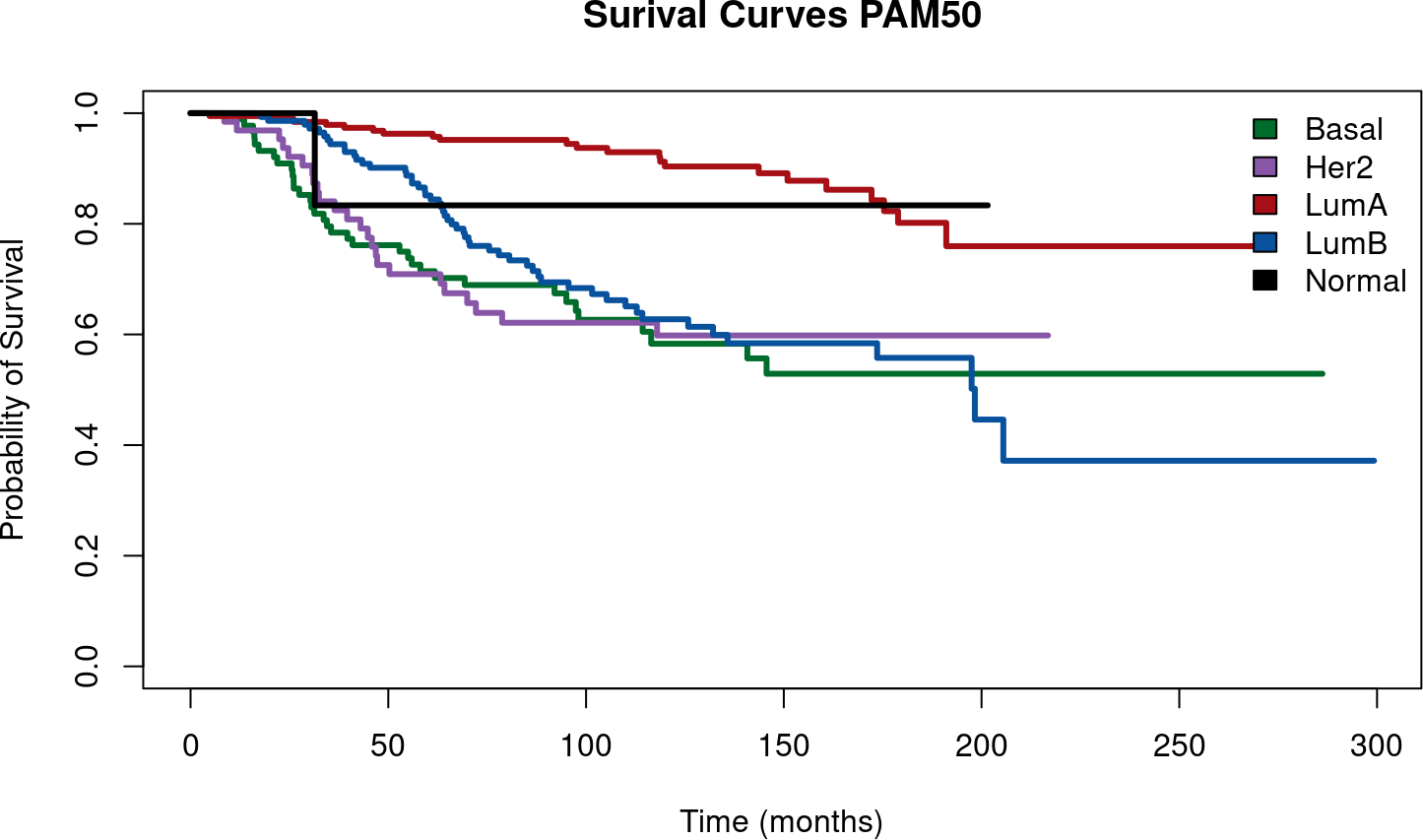``message("KAPLAN-MEIR CURVE - USING SCMOD2")``
``## KAPLAN-MEIR CURVE - USING SCMOD2``
``````plot(main = "Surival Curves SCMOD2", surv.obj.SCMOD2,
col =c("#006d2c", "#8856a7","#a50f15", "#08519c"),lty = 1,lwd = 3,
xlab = "Time (months)",ylab = "Probability of Survival")
legend("topright",
fill = c("#006d2c", "#8856a7","#a50f15", "#08519c"),
legend = c("Basal","Her2","LumA","LumB"),bty = "n")``````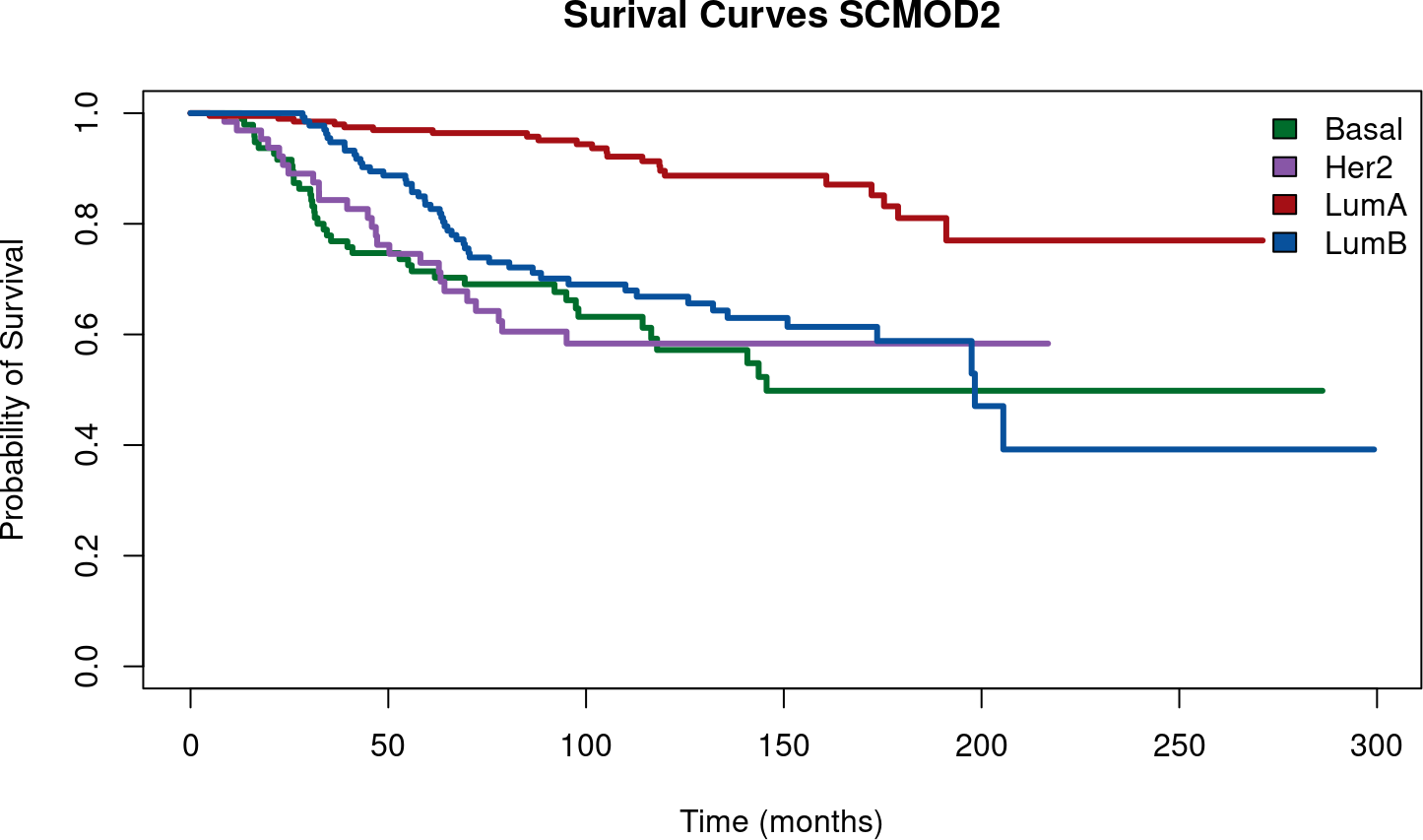``````## GENERATE A OVERLAYED PLOT OF SURVIVAL CURVES
message("Overlayed Surival Plots based on PAM50 and SCMOD2")``````
``## Overlayed Surival Plots based on PAM50 and SCMOD2``
``````                          ## Basal    Her2        LuminalA  LuminalB   Normal
plot(surv.obj.PAM50,col =c("#006d2c", "#8856a7","#a50f15", "#08519c", "#000000"),lty = 1,lwd = 3,
xlab = "Time (months)",ylab = "Probability of Survival",ymin = 0.2)
legend("topright",
fill = c("#006d2c", "#8856a7","#a50f15", "#08519c", "#000000"),
legend = c("Basal","Her2","LumA","LumB","Normal"),bty = "n")

par(new=TRUE)
## Basal    Her2        LuminalA  LuminalB
lines(surv.obj.SCMOD2,col =c("#006d2c", "#8856a7","#a50f15", "#08519c"),lwd=2,lty=5)
legend("bottomright",c("PAM50","SCMOD2"),lty=c("solid", "dashed"))``````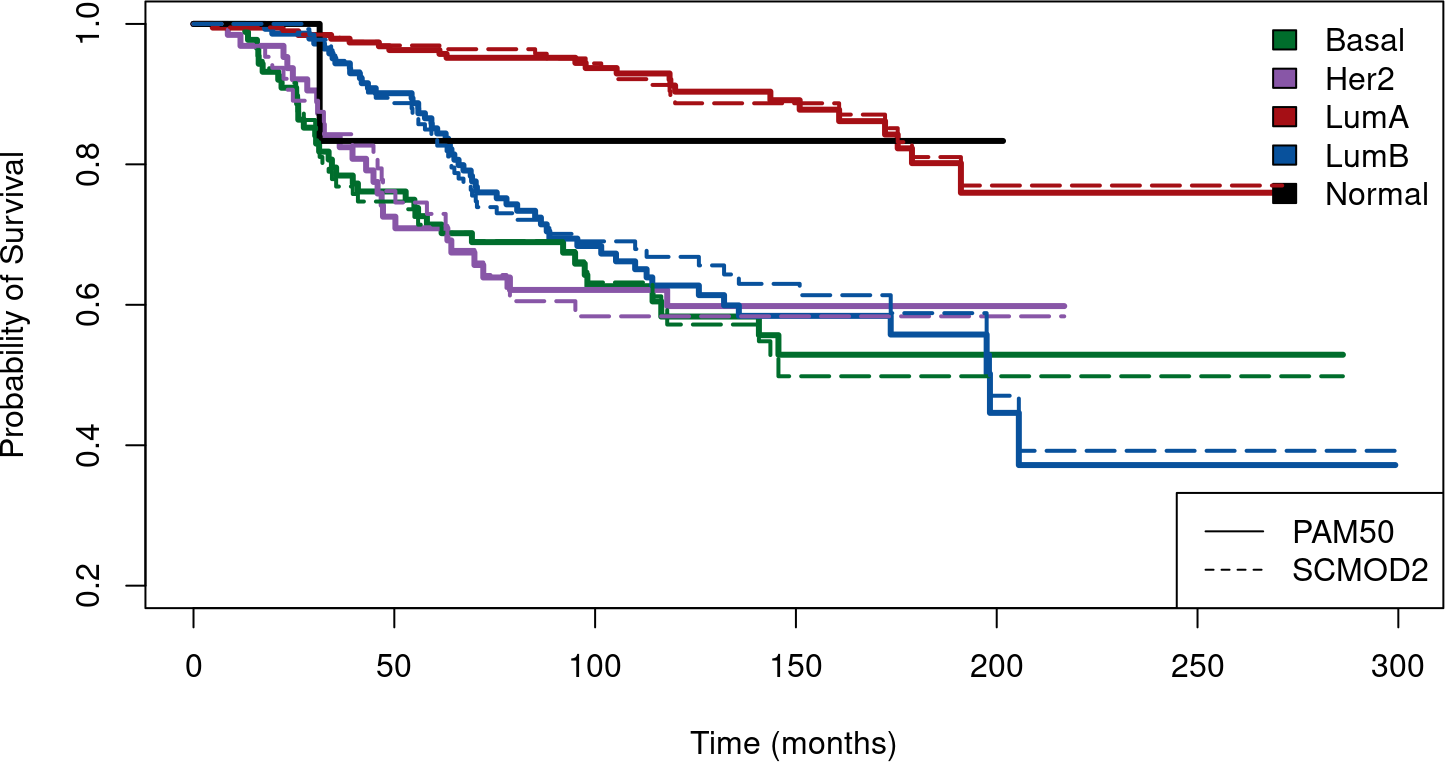We can now compare which of the molecular subtyping algorithms is more prognostic. To do this we use a Cross-validated Partial Likelihood (cvpl) calculation from survcomp. This returns the mean cross-validated partial likelihood, for each algorithm, using molecular subtypes for stratification

``````set.seed(12345)

PAM5_CVPL<-cvpl(x=data.for.survival.PAM50\$age,
surv.time=data.for.survival.PAM50\$months_to_death,
surv.event=data.for.survival.PAM50\$vital_status,
strata=as.integer(factor(data.for.survival.PAM50\$PAM50)),
nfold=10, setseed=54321)\$cvpl

SCMOD2_CVPL<-cvpl(x=data.for.survival.SCMOD2\$age,
surv.time=data.for.survival.SCMOD2\$months_to_death,
surv.event=data.for.survival.SCMOD2\$vital_status,
strata=as.integer(factor(data.for.survival.SCMOD2\$SCMOD2)),
nfold=10, setseed=54321)\$cvpl

print.data.frame(data.frame(cbind(PAM5_CVPL,SCMOD2_CVPL)))``````
``````##       PAM5_CVPL SCMOD2_CVPL
## logpl  1.424844    1.429175``````

## 4.2 Comparing Risk Prediction Models

We compute the risk scores using the following list of algorithms (and corresponding genefu functions):

• Subtype Clustering Model using just the AURKA gene: scmgene.robust()
• Subtype Clustering Model using just the ESR1 gene: scmgene.robust()
• Subtype Clustering Model using just the ERBB2 gene: scmgene.robust()
• NPI: npi()
• GGI: ggi()
• GENIUS: genius()
• EndoPredict: endoPredict()
• OncotypeDx: oncotypedx()
• TamR: tamr()
• GENE70: gene70()
• PIK3CA: pik3cags()
• rorS: rorS()
``````# Load gene signature
data(sig.endoPredict)
data(sig.oncotypedx)
data(sig.tamr13)
data(sig.gene70)
data(sig.pik3cags)
data(pam50)

dn <- c("transbig", "unt", "upp", "mainz", "nki")
dn.platform <- c("affy", "affy", "affy", "affy", "agilent")

res <- ddemo.all <- ddemo.coln <- NULL
for(i in 1:length(dn)) {

dd <- get(data(list=dn[i]))

#Extract expression set, pData, fData for each dataset
ddata <- t(exprs(dd))
ddemo <- phenoData(dd)@data
dannot <- featureData(dd)@data
ddemo.all <- c(ddemo.all, list(ddemo))
if(is.null(ddemo.coln))
{ ddemo.coln <- colnames(ddemo) } else
{ ddemo.coln <- intersect(ddemo.coln, colnames(ddemo)) }
rest <- NULL

## AURKA
## if affy platform consider the probe published in Desmedt et al., CCR, 2008
if(dn.platform[i] == "affy") { domap <- FALSE } else { domap <- TRUE }
modt <- scmgene.robust\$mod\$AURKA
## if agilent platform consider the probe published in Desmedt et al., CCR, 2008
if(dn.platform[i] == "agilent") {
domap <- FALSE
modt[ , "probe"] <- "NM_003600"
}
rest <- cbind(rest, "AURKA"=sig.score(x=modt, data=ddata, annot=dannot,
do.mapping=domap)\$score)

## ESR1
## if affy platform consider the probe published in Desmedt et al., CCR, 2008
if(dn.platform[i] == "affy") { domap <- FALSE } else { domap <- TRUE }
modt <- scmgene.robust\$mod\$ESR1
## if agilent platform consider the probe published in Desmedt et al., CCR, 2008
if(dn.platform[i] == "agilent") {
domap <- FALSE
modt[ , "probe"] <- "NM_000125"
}
rest <- cbind(rest, "ESR1"=sig.score(x=modt, data=ddata, annot=dannot,
do.mapping=domap)\$score)

## ERBB2
## if affy platform consider the probe published in Desmedt et al., CCR, 2008
if(dn.platform[i] == "affy") { domap <- FALSE } else { domap <- TRUE }
modt <- scmgene.robust\$mod\$ERBB2
## if agilent platform consider the probe published in Desmedt et al., CCR, 2008
if(dn.platform[i] == "agilent") {
domap <- FALSE
modt[ , "probe"] <- "NM_004448"
}
rest <- cbind(rest, "ERBB2"=sig.score(x=modt, data=ddata, annot=dannot,
do.mapping=domap)\$score)

## NPI
ss <- ddemo[ , "size"]
nn <- rep(NA, nrow(ddemo))
nn[complete.cases(ddemo[ , "node"]) & ddemo[ , "node"] == 0] <- 1
nn[complete.cases(ddemo[ , "node"]) & ddemo[ , "node"] == 1] <- 3
names(ss) <- names(gg) <- names(nn) <- rownames(ddemo)
rest <- cbind(rest, "NPI"=npi(size=ss, grade=gg, node=nn, na.rm=TRUE)\$score)

## GGI
if(dn.platform[i] == "affy") { domap <- FALSE } else { domap <- TRUE }
rest <- cbind(rest, "GGI"=ggi(data=ddata, annot=dannot,
do.mapping=domap)\$score)

## GENIUS
if(dn.platform[i] == "affy") { domap <- FALSE } else { domap <- TRUE }
rest <- cbind(rest, "GENIUS"=genius(data=ddata, annot=dannot,
do.mapping=domap)\$score)

## ENDOPREDICT
if(dn.platform[i] == "affy") { domap <- FALSE } else { domap <- TRUE }
rest <- cbind(rest, "EndoPredict"=endoPredict(data=ddata, annot=dannot,
do.mapping=domap)\$score)

# OncotypeDx
if(dn.platform[i] == "affy") { domap <- FALSE } else { domap <- TRUE }
rest <- cbind(rest, "OncotypeDx"=oncotypedx(data=ddata, annot=dannot,
do.mapping=domap)\$score)

## TamR
# Note: risk is not implemented, the function will return NA values
if(dn.platform[i] == "affy") { domap <- FALSE } else { domap <- TRUE }
rest <- cbind(rest, "TAMR13"=tamr13(data=ddata, annot=dannot,
do.mapping=domap)\$score)

## GENE70
# Need to do mapping for Affy platforms because this is based on Agilent.
# Hence the mapping rule is reversed here!
if(dn.platform[i] == "affy") { domap <- TRUE } else { domap <- FALSE }
rest <- cbind(rest, "GENE70"=gene70(data=ddata, annot=dannot, std="none",
do.mapping=domap)\$score)

## Pik3cags
if(dn.platform[i] == "affy") { domap <- FALSE } else { domap <- TRUE }
rest <- cbind(rest, "PIK3CA"=pik3cags(data=ddata, annot=dannot,
do.mapping=domap))

## rorS
# Uses the pam50 algorithm. Need to do mapping for both Affy and Agilent
rest <- cbind(rest, "rorS"=rorS(data=ddata, annot=dannot,
do.mapping=TRUE)\$score)

## GENE76
# Mainly designed for Affy platforms. Has been excluded here

# BIND ALL TOGETHER
res <- rbind(res, rest)
}
names(ddemo.all) <- dn``````

For further analysis and handling of the data we store all information in one object. We also remove the duplicated patients from the analysis and take only those patients into account, that have complete information for nodal, survival and treatment status.

``````ddemot <- NULL
for(i in 1:length(ddemo.all)) {
ddemot <- rbind(ddemot, ddemo.all[[i]][ , ddemo.coln, drop=FALSE])
}
res[complete.cases(ddemot[ ,"dataset"]) & ddemot[ ,"dataset"] == "VDX", "GENIUS"] <- NA

## select only untreated node-negative patients with all risk predictions
## ie(incomplete cases (where risk prediction may be missing for a sample) are subsequently removed))
# Note that increasing the number of risk prediction analyses
# may increase the number of incomplete cases
# In the previous vignette for genefu version1, we were only testing 4 risk predictors,
# so we had a total of 722 complete cases remaining
# Here, we are now testing 12 risk predictors, so we only have 713 complete cases remaining.
# The difference of 9 cases between the two versions are all from the NKI dataset.
myx <- complete.cases(res, ddemot[ , c("node", "treatment")]) &
ddemot[ , "treatment"] == 0 & ddemot[ , "node"] == 0 & !is.element(rownames(ddemot), duPL)

res <- res[myx, , drop=FALSE]
ddemot <- ddemot[myx, , drop=FALSE]``````

To compare the risk score performances, we compute the concordance index, which is the probability that, for a pair of randomly chosen comparable samples, the sample with the higher risk prediction will experience an event before the other sample or belongs to a higher binary class.

``````cc.res <- complete.cases(res)
datasetList <- c("MAINZ","TRANSBIG","UPP","UNT","NKI")
riskPList <- c("AURKA","ESR1","ERBB2","NPI", "GGI", "GENIUS",
"EndoPredict","OncotypeDx","TAMR13","GENE70","PIK3CA","rorS")
setT <- setE <- NULL
resMatrix <- as.list(NULL)

for(i in datasetList)
{
dataset.only <- ddemot[,"dataset"] == i
patientsAll <- cc.res & dataset.only

## set type of available survival data
if(i == "UPP") {
setT <- "t.rfs"
setE <- "e.rfs"
} else {
setT <- "t.dmfs"
setE <- "e.dmfs"
}

# Calculate cindex computation for each predictor
for (Dat in riskPList)
{
cindex <- t(apply(X=t(res[patientsAll,Dat]), MARGIN=1, function(x, y, z) {
tt <- concordance.index(x=x, surv.time=y, surv.event=z, method="noether", na.rm=TRUE);
return(c("cindex"=tt\$c.index, "cindex.se"=tt\$se, "lower"=tt\$lower, "upper"=tt\$upper)); },
y=ddemot[patientsAll,setT], z=ddemot[patientsAll, setE]))

resMatrix[[Dat]] <- rbind(resMatrix[[Dat]], cindex)
}
}``````

Using a random-effects model we combine the dataset-specific performance estimated into overall estimates for each risk prediction model:

``````for(i in names(resMatrix)){
#Get a meta-estimate
ceData <- combine.est(x=resMatrix[[i]][,"cindex"], x.se=resMatrix[[i]][,"cindex.se"], hetero=TRUE)
cLower <- ceData\$estimate + qnorm(0.025, lower.tail=TRUE) * ceData\$se
cUpper <- ceData\$estimate + qnorm(0.025, lower.tail=FALSE) * ceData\$se

cindexO <- cbind("cindex"=ceData\$estimate, "cindex.se"=ceData\$se, "lower"=cLower, "upper"=cUpper)
resMatrix[[i]] <- rbind(resMatrix[[i]], cindexO)
rownames(resMatrix[[i]]) <- c(datasetList, "Overall")
}``````

In order to compare the different risk prediction models we compute one-sided p-values of the meta-estimates:

``````pv <- sapply(resMatrix, function(x) { return(x["Overall", c("cindex","cindex.se")]) })
pv <- apply(pv, 2, function(x) { return(pnorm((x - 0.5) / x, lower.tail=x < 0.5)) })
printPV <- matrix(pv,ncol=length(names(resMatrix)))
rownames(printPV) <- "P-value"
colnames(printPV) <- names(pv)
printPV<-t(printPV)``````

And print the table of P-values:

``knitr::kable(printPV, digits=c(0, -1))``
P-value
AURKA 0
ESR1 0
ERBB2 0
NPI 0
GGI 0
GENIUS 0
EndoPredict 0
OncotypeDx 0
TAMR13 0
GENE70 0
PIK3CA 0
rorS 0

The following figures represent the risk score performances measured by the concordance index each of the prognostic predictors.

``````RiskPList <- c("AURKA","ESR1","ERBB2","NPI", "GGI", "GENIUS",
"EndoPredict","OncotypeDx","TAMR13","GENE70","PIK3CA","rorS")
datasetListF <- c("MAINZ","TRANSBIG","UPP","UNT","NKI", "Overall")
myspace <- "   "
par(mfrow=c(2,2))
for (RP in RiskPList)
{

#<<forestplotDat,fig=TRUE>>=
## Forestplot
tt <- rbind(resMatrix[[RP]][1:5,],
"Overall"=resMatrix[[RP]][6,])

tt <- as.data.frame(tt)
labeltext <- (datasetListF)

r.mean <- c(tt\$cindex)
r.lower <- c(tt\$lower)
r.upper <- c(tt\$upper)

metaplot.surv(mn=r.mean, lower=r.lower, upper=r.upper, labels=labeltext, xlim=c(0.3,0.9),
boxsize=0.5, zero=0.5,
col=meta.colors(box="royalblue",line="darkblue",zero="firebrick"),
main=paste(RP))

}``````